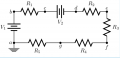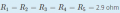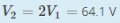# Thevenin equivalent resistance

#### AnaLI

Joined Nov 8, 2016
2
Hey, guys,
I'm having a lot of trouble understanding the solution to this exercise:The goal is to calculate the Thévenin resistance between e and f. The solution is 2.3 ohm. WHY??
I'm pretty sure it's 2.9 ohm.

If someone understands why it is 2.3ohm, can you explain, please? Thanks.

#### WBahn

Joined Mar 31, 2012
26,398
How are you arriving at 2.9 Ω?

If you zero the sources, what are you left with looking between points e and f?

Don't you have 2.9 Ω in parallel with some other resistance? So isn't the equivalent resistance of two resistances always less than the smaller of the two?

#### AnaLI

Joined Nov 8, 2016
2
How are you arriving at 2.9 Ω?

If you zero the sources, what are you left with looking between points e and f?

Don't you have 2.9 Ω in parallel with some other resistance? So isn't the equivalent resistance of two resistances always less than the smaller of the two?
You're saying I am to consider the R3 in parallel with (R1+R2+R4+R5)?
Ok, yes, that yields 2.3ohm. I get way too confused when there are ground connections involved...

Thank you, I'm sorry for the trouble. Guess this was a really silly question.

#### Bordodynov

Joined May 20, 2015
2,939
X=(R1+R2+R5+R4)||R3=2.32
Move seats R1 and V2 (the current through R3 will not change). Unite voltage sources. Transfer the ground to the point f (current through R3 will not change). Move the resistors and source. Get the source and four series resistors. Make the conversion and get my formula.

#### WBahn

Joined Mar 31, 2012
26,398
You're saying I am to consider the R3 in parallel with (R1+R2+R4+R5)?
Ok, yes, that yields 2.3ohm. I get way too confused when there are ground connections involved...

Thank you, I'm sorry for the trouble. Guess this was a really silly question.
The ground connection in this case is a complete red herring since nothing of interest is referred to the ground, so it can be ignored.

#### Dodgydave

Joined Jun 22, 2012
10,088
11.6 ohms in parallel with 2.9 ohms = 2.32 ohms.....